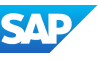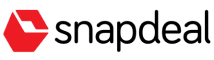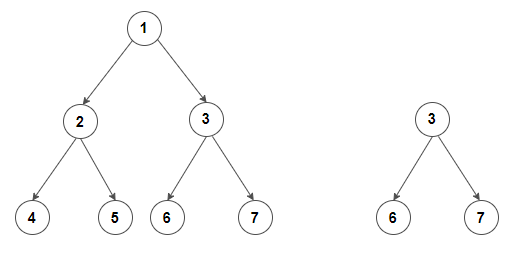New update is available. Click here to update.
Topics

# Subtree of Another Tree

Easy0/40
Average time to solve is 10m+14 more companies

## Problem statement

Given two binary trees T and S, check whether tree S has exactly the same structure and node values with a subtree of T, i.e., check if tree S is a subtree of the tree T.

A subtree of a tree T is a tree S consisting of a node in T and all of its descendants in T. The subtree corresponding to the root node is the entire tree; the subtree corresponding to any other node is called a proper subtree.

Detailed explanation ( Input/output format, Notes, Images )
Constraints:
``````1 <= T <= 100
1 <= N, M <= 1000
0 <= data <= 10^6 and data != -1

Where ‘T’ is the number of test cases, ‘N’ and ‘M’ are the number of nodes in the given binary trees’, and “data” is the value of the binary tree node.

Time Limit: 1 sec.
``````
Sample Input 1:
``````2
1 2 3 4 5 6 7 -1 -1 -1 -1 -1 -1 -1 -1
3 6 7 -1 -1 -1 -1
3 4 5 1 2 -1 -1 -1 -1 0 -1 -1 -1
4 1 2 -1 -1 -1 -1
``````
Sample Output 1:
``````true
false
``````
Explanation of Sample Input 1:``````For the first test case, the second tree is a subtree of the first tree as the second tree has exactly the same structure and node values with a subtree of the first tree.

For the second test case, the second tree is not a subtree of the first tree because node 2 in the first tree has 1 child having a value 0, whereas node 2 in the second tree doesn’t have any child.
``````
Sample Input 2:
``````2
26 10 3 4 6 -1 3 -1 30 -1 -1 -1 -1 -1 -1
10 4 6 -1 30 -1 -1 -1 -1
3 4 5 1 2 -1 -1 -1 -1 -1 -1
4 1 -1 -1 -1
``````
Sample Output 2:
``````true
false
``````Console